﻿• 价格透明
• 信息保密
• 进度掌控
• 售后无忧# 什么是张量

Tensor:(向量)

0标量s=1
1向量s=[1,2,3,4,5]
2矩阵s=[ [1,2,3 ], [4,5,6 ] ]
n张量s=[ [ [ [… ] ] ] ]

# 数据类型：

``````tf.int/tf.float:
tf.int32,	tf.int64,	tf.float32,		tf.float64
tf.bool:
tf.constant([True,False])//tf.constant()是申明一个常量
tf.string：
tf.constant("I Love You")
``````

# 创建一个Tensor:

``````tf.constant(张量内容，dtype=数据类型)
``````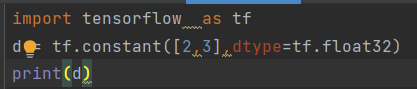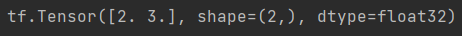eg:将numpy的数据转化为Tensor类型：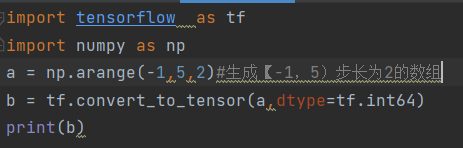1. `tf.zeros(维度)`:创建一个全为0的张量。
2. `tf.ones(维度)`:创建一个全为1的张量。
3. `tf.fill(维度，指定值)`:将这个张量全部设置为指定值。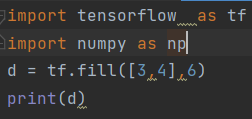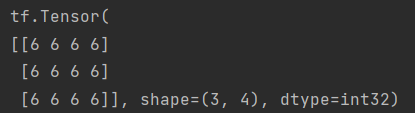4. 随机生成张量：
``````import tensorflow  as tf
import numpy as np
#随机生成3行4列的张量，里面的元素符合以1为均值，0.1为标准差的数据：
d = tf.random.normal([3,4],mean=1,stddev=0.1)

#随机生成2行2列的张量，里面的元素最小值为1，最大值为2:
f = tf.random.uniform([2,2],minval=1,maxval=2)

print(d)
print(f)
``````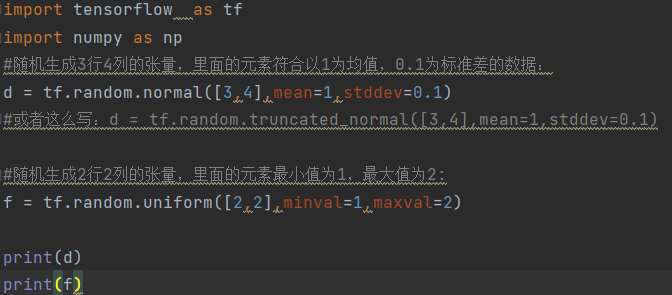### 低价透明### 金牌服务### 信息保密